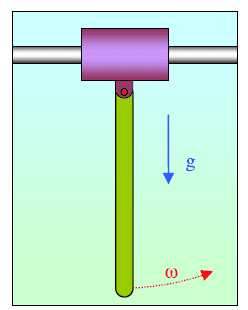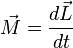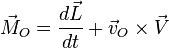# Physical pendulum with no fixed pole1. Homework Statement

The violet sleeve has mass M and is free to move horizontally without friction. The green rod has mass 2M and length L and can rotate around a pivot on the sleeve without friction. At t=0, the rod is in vertical position and is rotating with angular velocity w. What are the physical quantities that are constant? What is the maximum angular amplitude of oscillations and maximum sleeve speed?

2. The attempt at a solution
K_{tot} + U_{tot} = constant (there aren't dissipative forces such as friction)
Linear momentum: there are external forces (weight force and vincular reaction) so it isn't constant.
Angular momentum: whatever pole I choose there is some external force that have nonzero torque, so it isn't constant.

I tried to solve the problem calculating the torque with respect to the pivot, but the problem is: that pole is in motion, so I don't have, but I have(pole velocity isn't parallel to mass center velocity)... and so I can't go on to calculate angular acceleration and I'm stuck.

Could you help me?

Orodruin
Staff Emeritus
Homework Helper
Gold Member
2021 Award
Are you familiar with Lagrange mechanics?

TSny
Homework Helper
Gold Member
What can you say about the horizontal component of the net external force on the system?

@Orodruin: no, I don't know anything about it.
@TSny: There's no net horizontal force, hence the horizontal linear momentum is constant.. but how can I proceed then?

TSny
Homework Helper
Gold Member
@TSny: There's no net horizontal force, hence the horizontal linear momentum is constant.
Yes.
.. but how can I proceed then?
Consider the system at the instant θ is a maximum.

Yes.

Consider the system at the instant θ is a maximum.
I think I've got the idea. But there's a doubt. At theta maximum, the rod has zero angular velocity, but with respect to the pole (so the system is all going with velocity V)... So i can extract from there the maximum sleeve velocity... Am I wrong?

TSny
Homework Helper
Gold Member
I think I've got the idea. But there's a doubt. At theta maximum, the rod has zero angular velocity, but with respect to the pole (so the system is all going with velocity V)
Yes, at max θ the rod has zero angular speed. There is no need to say "with respect to the pole".
And, yes, all points of the rod and sleeve have the same velocity at this instant.
... So i can extract from there the maximum sleeve velocity...
Does the sleeve have its maximum speed at the instant that θ is a maximum?

Yes, at max θ the rod has zero angular speed. There is no need to say "with respect to the pole".
And, yes, all points of the rod and sleeve have the same velocity at this instant.
I don't understand "There is no need to say "with respect to the pole". ". Well, I think it's important that the rod points speed is zero with respect to the pole, so that sleeve and rod have the same velocity. (Now I'm thinking you were pointing out that I said angular velocity respect to the pole, but I was thinking at corresponding linear velocities of the points).
Does the sleeve have its maximum speed at the instant that θ is a maximum?
I think so, but I can't show why.

Thanks a lot for your support!

(I got a bit confused about my assumptions after seeing this animation https://upload.wikimedia.org/wikipedia/commons/4/4d/Pendulum_movable_support.gif but maybe it's just a different problem)

Orodruin
Staff Emeritus
Homework Helper
Gold Member
2021 Award
I think so, but I can't show why.

Consider the case where the total linear momentum of the entire contraption is zero ...

TSny
Homework Helper
Gold Member
(Now I'm thinking you were pointing out that I said angular velocity respect to the pole, but I was thinking at corresponding linear velocities of the points).
Yes. I was pointing out that angular velocity does not need to be specified relative to some point.

The sleeve does not have its max speed when θ is max. The first question is to find θmax. You know that all points of the system have the same velocity when θ = θmax. See if you can use this fact along with the conservation laws to determine θmax.
(I got a bit confused about my assumptions after seeing this animation https://upload.wikimedia.org/wikipedia/commons/4/4d/Pendulum_movable_support.gif but maybe it's just a different problem)
This animation shows the same system but with different initial conditions. Or, you can think of the animation as showing your system from a particular moving frame of reference. Going to this frame of reference might be helpful for finding the max speed of the sleeve.

[EDIT: After watching the animation more closely, it appears that there's frictional damping in the system. So, it's not quite the same system. Nevertheless, you might want to consider a frame of reference where the total linear momentum of your system is zero. But, it's not necessary to do this.]

Last edited:
@TSny ok I followed your hints and I found cos (thetamax) from the conservation of energy (at t=0 the energy is all rotational at thetamax the energy is gravitational and translational (i can find the speed from the conservation of horizontal linear momentum). Now I can't go on with Max sleeve velocity, at first I supposed it was at thetamax, you pointed out i was wrong.. and I can't guess in which position it is!

@Orodruin sorry but I can't get the point :(

Orodruin
Staff Emeritus
Homework Helper
Gold Member
2021 Award
sorry but I can't get the point :(

It is a simple argument for why your statement is false. In that situation, the velocity of the sleeve is zero when ##\theta## is maximal.

It is a simple argument for why your statement is false. In that situation, the velocity of the sleeve is zero when ##\theta## is maximal.
Why is that? When theta is max the pendulum is quiet with respect to the pole so it's moving at the sleeve velocity.. Why is the total linear momentum zero?

P.s. could you explain me what is the configuration in which the sleeve velocity is maximum? I just can't see it!

Orodruin
Staff Emeritus
Homework Helper
Gold Member
2021 Award
Why is that? When theta is max the pendulum is quiet with respect to the pole so it's moving at the sleeve velocity.. Why is the total linear momentum zero?

If the pendulum is at relative rest to the sleeve and the total momentum is zero, what is the velocity of the sleeve (and hence also the pendulum)?

If the pendulum is at relative rest to the sleeve and the total momentum is zero, what is the velocity of the sleeve (and hence also the pendulum)?
I'm tempted of replying "sleeve-pendulum velocity is zero" but i'm still not sure why at theta max the total linear momentum is 0 .. :(
Moreover, i still can't see how this argument could help me in finding sleeve max velocity..

P.s. what about the reasoning to find theta max that I summerized in a previous message? Is it correct?

TSny
Homework Helper
Gold Member
@TSny ok I followed your hints and I found cos (thetamax) from the conservation of energy (at t=0 the energy is all rotational at thetamax the energy is gravitational and translational (i can find the speed from the conservation of horizontal linear momentum).
Sounds good.

Now I can't go on with Max sleeve velocity, at first I supposed it was at thetamax, you pointed out i was wrong.. and I can't guess in which position it is!

Consider what happens after the rod reaches max angle to the right. As the rod begins to swing back down toward a vertical position, what happens to the horizontal momentum of the rod? So what happens to the horizontal momentum of the sleeve as the rod swings back down?

TSny
Homework Helper
Gold Member
but i'm still not sure why at theta max the total linear momentum is 0
In the frame of reference of the picture given in the problem, the total linear momentum is not zero. However, you can imagine going to a moving inertial frame in which the total momentum is zero. You can work out the max speed of the sleeve in this frame and then transform back to the original frame.

But
In the frame of reference of the picture given in the problem, the total linear momentum is not zero. However, you can imagine going to a moving inertial frame in which the total momentum is zero. You can work out the max speed of the sleeve in this frame and then transform back to the original frame.
But why the sleeve velocity is maximum at theta max?

Orodruin
Staff Emeritus
Homework Helper
Gold Member
2021 Award
But

But why the sleeve velocity is maximum at theta max?
It isn't.

It isn't.
So I have misinterpreted @TSny message. Let's start again. I choose the frame of reference in which total linear momentum is zero when theta is max. And then? I can't see how to "work out the max speed of the sleeve in this frame" !

@TSny "Consider what happens after the rod reaches max angle to the right. As the rod begins to swing back down toward a vertical position,what happens to the horizontal momentum of the rod?" Well, I think that the rod velocity with respect to the pole has decreased up to zero (in theta max) and then it reverse sign and starts to increase again... But this is only with respect to the pole and this fact makes me impossible to get how things are going. Could you please be more explicit? I'm stuck with it and cannot go on.

Orodruin
Staff Emeritus
Homework Helper
Gold Member
2021 Award
I choose the frame of reference in which total linear momentum is zero when theta is max. And then? I can't see how to "work out the max speed of the sleeve in this frame" !
There is no need to specify "when theta is max". As you have already concluded, the linear momentum is conserved. Think about how you can use this fact to your advantage. What does it mean that the linear momentum is zero?

Edit: Note that both the sleeve and the rod will move in this reference frame!

•bznm
There is no need to specify "when theta is max". As you have already concluded, the linear momentum is conserved. Think about how you can use this fact to your advantage. What does it mean that the linear momentum is zero?

Edit: Note that both the sleeve and the rod will move in this reference frame!
I think you are saying that it means that the two moments are opposite. So I get the maximum (in absolute value) momentum for the sleeve when the rod momentum is maximum (in absolute value). Is it correct? But in that frame, the rod momentum is maximum when?

Orodruin
Staff Emeritus
Homework Helper
Gold Member
2021 Award
Have you tried writing down expressions for the momenta and energy?

well, I am not used to write these expressions in particular frame:
Mv (current sleeve velocity)= 2Mw_cm, where w_cm is w(the current angular velocity)*L/3 (the distance between the pole and the center of mass) .. I equate them because the sum should be 0
E=1/2 M v^2+1/2 I(the moment of inertia)*w^2+2M*g*(L-L*cos theta)

I'm pretty sure that's wrong, but please understand me: it's a very unusual exercise for me!

TSny
Homework Helper
Gold Member
@TSny "Consider what happens after the rod reaches max angle to the right. As the rod begins to swing back down toward a vertical position,what happens to the horizontal momentum of the rod?" Well, I think that the rod velocity with respect to the pole has decreased up to zero (in theta max) and then it reverse sign and starts to increase again... But this is only with respect to the pole and this fact makes me impossible to get how things are going. Could you please be more explicit? I'm stuck with it and cannot go on.

In the original frame of reference, when the rod reaches maximum angle, the rod and sleeve are moving together with some speed V toward the right. As the rod swings downward, what happens to the horizontal component of the velocity, vx, of the center of mass of the rod relative to this frame of reference? Does vx increase, decrease, or stay at the value V?

If it's difficult to see the answer to this question, it is helpful to think about it from the point of view of the reference frame that moves with constant speed to the right such that the total linear momentum of the system is zero. In this frame, think about what the system is doing at max angle of the rod. Then think about what must happen as the rod swings back down.

In the original frame of reference, when the rod reaches maximum angle, the rod and sleeve are moving together with some speed V toward the right. As the rod swings downward, what happens to the horizontal component of the velocity, vx, of the center of mass of the rod relative to this frame of reference? Does vx increase, decrease, or stay at the value V?
It should increase because when it swings down some potential energy becomes kinetic... Is it wrong?

TSny
Homework Helper
Gold Member
Does the swinging down contribute velocity to the right or to the left for the CM of the rod?

Does the swinging down contribute velocity to the right or to the left for the CM of the rod?
Well, I think left, because it goes towards the vertical position... so vx decreases..

TSny
Homework Helper
Gold Member
Yes. At max height, the rod has some momentum, px, to the right (relative to the original reference frame). As it swings down, the velocity of the CM picks up some contribution to the left due to the rotational motion. That is, there is a change in velocity of the CM of the rod toward the left. So, taking x to be positive toward the right, does px for the rod increase or decrease as it swings down?

Well, I think left, because it goes towards the vertical position...
Yes. At max height, the rod has some momentum, px, to the right (relative to the original reference frame). As it swings down, the velocity of the CM picks up some contribution to the left due to the rotational motion. That is, there is a change in velocity of the CM of the rod toward the left. So, taking x to be positive toward the right, does px for the rod increase or decrease as it swings down?
I think that the rod momentum decreases while the sleeve momentum increases, because the horizontal momentum must be constant...

TSny
Homework Helper
Gold Member
You're on a skateboard holding a ball and moving toward the right. You and the ball are moving together with a speed V. You then throw the ball toward the left so that the ball has a change in velocity toward the left. Taking x as positive toward the right, does the change in velocity of the ball cause px for the ball to increase or decrease? What about your momentum, Px? Does it increase or decrease?.

px for the ball decreases while px for me increases.. I think that you missed my previous post edit, I'm sorry..

TSny
Homework Helper
Gold Member
px for the ball decreases while px for me increases.. I think that you missed my previous post edit, I'm sorry..
Oops, I did miss it. OK, good. So, the momentum of the sleeve increases toward the right as the rod swings down. So, does the sleeve have maximum speed when the rod has its maximum angle?

Oops, I did miss it. OK, good. So, the momentum of the sleeve increases toward the right as the rod swings down. So, does the sleeve have maximum speed when the rod has its maximum angle?
Well, It doesn't seem so to me now... I think that while the rod is going down,the sleeve gains horizontal momentum... Is it that the maximum sleeve velocity is when the rod has come back in the vertical position?

TSny
Homework Helper
Gold Member
Well, It doesn't seem so to me now... I think that while the rod is going down,the sleeve gains horizontal momentum...
Yes
Is it that the maximum sleeve velocity is when the rod has come back in the vertical position?
Here's where the zero-momentum reference frame is useful. The animation you posted earlier is similar to being in the zero-momentum frame. What is the orientation of the rod when the sleeve has maximum speed?